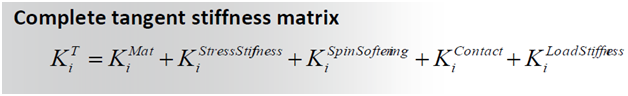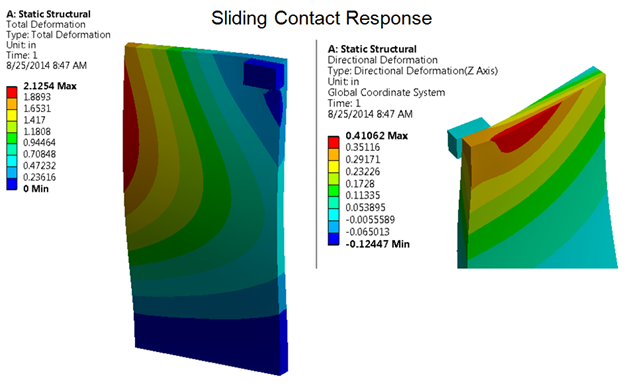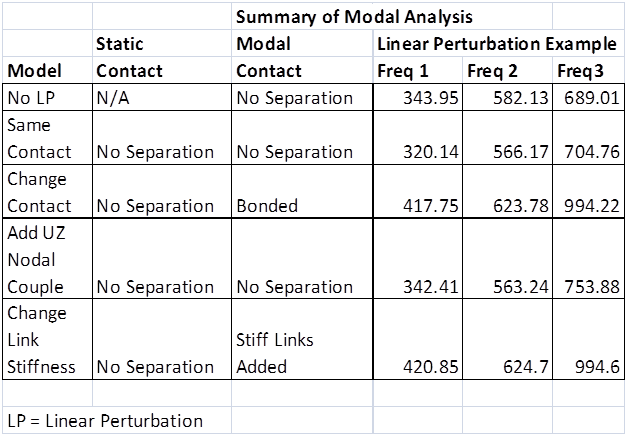you are here:/ News & Insights / Engineering Advantage Blog / Improve the Accuracy of Your Modal Analysis Using Linear Perturbation

# Improve the Accuracy of Your Modal Analysis Using Linear PerturbationOctober 3, 2014 By: Peter Barrett

Are you interested in improving the accuracy of your linear dynamics or buckling studies? Linear perturbation captures the results of nonlinear static analyses to be used as initial conditions in modal or buckling eigenvalue analyses. In theory, a linear perturbation analysis can be applied to any finite element analysis type, but it is most often utilized in pre-stressed modal analyses. To get started, a restart procedure is used to assess the prior “base” nonlinear stiffness. Key points about the typical procedure:

• Geometry is updated into the “deformed configuration”
• Material stiffness is updated to the tangent stiffness stiffness prior to the subsequent linear analysis.
• Contact elements can take the restart status or can be user-modified.  For example, you can convert nonlinear frictional contact to a bonded response.
• Common applications include initial conditions for modal, buckling and harmonic analyses.  The eigenvalue extraction procedures are unchanged; the only difference is the reformulation of the stiffness and mass matrices based on the prior analyses.

Figure 1 above illustrates how the tangent stiffness matrix from the prior solution (the base analysis) is obtained for the linear perturbation analysis. The stiffness represents a "snap-shot" of the base nonlinear stiffness which is generally obtained from restart files.

This matrix regeneration is the first phase of a linear perturbation procedure, where the stiffness is stored in a “frozen” state. No further change in stiffness occurs in the buckling, harmonic or modal analysis. Components of the "frozen" stiffness matrices are illustrated in Figure 2 and can include:

• Large deflection effects including the load stiffening matrix introduced by external pressure loads or by follower force effects.
• Tangent material property stiffness
• Elastic stiffness for plastic material models
• Current contact status  (This can also be changed later.)Once the stiffness is defined, additional input or modeling changes can also be incorporated prior to the modal analysis.

Why would you want to change the local stiffness between the static and modal analyses?

• Connections can sometimes give natural frequency results that are too high or too low compared to experimental data. Even for welded structures, one must sometimes tune the resulting stiffness such that the model matches lab data.
• There is extensive precedent for using joint tuning factors to account for effects of stiffness, friction, and damping at joints. Changing contact properties is one way to account for these stiffness differences.
• The best stiffness value to use will vary with geometry, loading, mesh size, etc. So, there is no firm guidance here, but the impact on natural frequencies can be significant, thus having the ability to further "tweak" the stiffness is a valuable method to calibrate with test data.

Linear Perturbation can easily be used to adjust materials, constraints and connection stiffnesses between the static and modal analyses to account for these connection anomalies.

The effects of using linear perturbation with added contact, material and coupling equation modeling changes are demonstrated on a simple plate/block model (illustrated in Figure 3).  The connection between the block and plate is further modified after the tangent stiffness is read, but before the modal analysis is performed.Table 1 demonstrates the significant impact of these contact, coupling and material modeling changes on the first three natural frequencies for the plate-block example.  The fundamental  frequency ranges from 320 to 420 Hz as a result of minor connection modeling changes (in addition to pre-stress).  For example, in row four of Table 1, adding UZ (lateral) coupling of a single nodal point between the block and the plate increases the first frequency from 320 to 340 Hz.

The stiff link material modeling change method demonstrated utilizes a series of "weak" links in the static model that were stiffened by increasing the modulus after the nonlinear static analysis to create more of a rigid bond between the plate and block hence mimicking the conversion from no separation to bonded contact. Intermediate link material stiffness values can be used for calibration to test where the connection stiffness is reduced.The linear perturbation method is useful to account for stiffness changes between the static and dynamic analyses.  In addition to updating the geometry modifying contact settings, changing couples or constraint equations or changing material properties can result in better correlation with experimental data.

I am curious if others have utilized linear perturbation methods in a similar manner?  Has anyone had experience with modeling changes to the local FEM stiffness between the static and modal analyses to better calibrate their analytical models with physical tests?